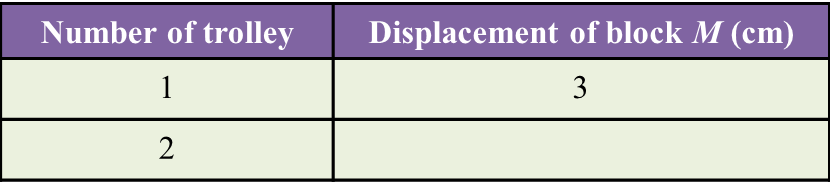# 5.7.1 The Concept of Momentum (Structured Questions)

Question 1:
Diagram 1.1 and Diagram 1.2 show an experiment to study the collision between trolleys of different masses with a plasticine block.Both trolleys move with the same velocity.
(a) State the variables in this experiment.
(i) Manipulated variable [1 mark]
(ii) Responding variable [1 mark]

(b)(i) What can you observe about the plasticine block after the collision? [1 mark]
(ii) State one inference that can be made based on the observation in (b)(i). [1 mark]

(c) State the hypothesis of this experiment. [1 mark]

(a)(i)
Mass of the trolley

(a)(ii)
Depth of the dent on the plasticine block

(b)(i)

Trolley T produced a deeper dent on the plasticine block compared to the dent produced by trolley S after their collision with the plasticine block.

(b)(ii)

When a moving trolley with a bigger mass collides with a plasticine block, it produces a deeper dent on the plasticine block.

(c)
The bigger the mass of a moving trolley which collides with a plasticine block, the deeper the dent which will be formed on the plasticine block.

Question 2:
Diagram 2.1 shows an experiment to study the collision of a trolley with block M. After collision, block M is displaced to a new position.
The experiment is repeated using two trolleys as shown in Diagram 2.2.The result of this experiment is shown in Table below.(a) Measure the displacement of block M after the collision in Diagram 2.2.  [1 mark]

(b) State one observation about displacement of block M in diagram 2.1 and 2.2[1 mark]

(c) State the variables in this experiment.
(i) Constant variable [1 mark]
(ii) Manipulated variable [1 mark]

(d) Predict the displacement of block M if three trolleys are used. [1 mark]